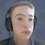# Vigenère Decipher

  1 2 3 4 5 6 7 8 9 10 11 12 13 14 15 16 17 18 19 20 21 22 23 24 25 26 27 28 29 30 31 32 33 34 35 36 37 38 39 40 41 42 43 44 45 46 import math import re def mostFrequent(List): ##Useful later on return max(set(List), key = List.count) def listToString(s): ##Useful later on str1 = " " return (str1.join(s)) Alphabet = ["A", "B", "C", "D", "E", "F", "G", "H", "I", "J", "K", "L", "M", "N", "O", "P", "Q", "R", "S", "T", "U", "V", "W", "X", "Y", "Z"] ##Generally needed Alphabet2 = ["A", "B", "C", "D", "E", "F", "G", "H", "I", "J", "K", "L", "M", "N", "O", "P", "Q", "R", "S", "T", "U", "V", "W", "X", "Y", "Z", "A", "B", "C", "D", "E", "F", "G", "H", "I", "J", "K", "L", "M", "N", "O", "P", "Q", "R", "S", "T", "U", "V", "W", "X", "Y", "Z"] ##Needed to avoid problems with the .index function ST = [] ##SecretText Key = [] ##Key used to encode with the Vigènere Cipher PT = [] ##PublicText tmp = [] ##Temporary list to analyse frequency of occurence of letters def main(file, Keylength): with open(file, 'r') as f: text = f.read().strip().split() len_chars = sum(len(word) for word in text) ##len_chars needed for future loops with open(file) as fileobj: for line in fileobj: for ch in line: ST.append(ch) ##SecretText into list for i in range(0, Keylength): for i in range(i, len_chars, Keylength): tmp.append(ST[i]) ##every i-th char decoded with the same letter in the Key diff = Alphabet.index(mostFrequent(tmp)) - Alphabet.index("E") ##standard frequency of occurence analysis Key.append(Alphabet[diff]) del tmp[:] n = 0 i = 0 while i < len_chars: if n < Keylength: a = Alphabet.index(Key[n]) b = Alphabet.index(ST[i]) c = b - a d = c % 26 PT.append(Alphabet2[d]) ##Reversing Vigenère n += 1 i += 1 else: n -= 5 print(listToString(PT))Note by Will Schefner
1 month ago

This discussion board is a place to discuss our Daily Challenges and the math and science related to those challenges. Explanations are more than just a solution — they should explain the steps and thinking strategies that you used to obtain the solution. Comments should further the discussion of math and science.

When posting on Brilliant:

• Use the emojis to react to an explanation, whether you're congratulating a job well done , or just really confused .
• Ask specific questions about the challenge or the steps in somebody's explanation. Well-posed questions can add a lot to the discussion, but posting "I don't understand!" doesn't help anyone.
• Try to contribute something new to the discussion, whether it is an extension, generalization or other idea related to the challenge.
• Stay on topic — we're all here to learn more about math and science, not to hear about your favorite get-rich-quick scheme or current world events.

MarkdownAppears as
*italics* or _italics_ italics
**bold** or __bold__ bold
- bulleted- list
• bulleted
• list
1. numbered2. list
1. numbered
2. list
Note: you must add a full line of space before and after lists for them to show up correctly
paragraph 1paragraph 2

paragraph 1

paragraph 2

[example link](https://brilliant.org)example link
> This is a quote
This is a quote
    # I indented these lines
# 4 spaces, and now they show
# up as a code block.

print "hello world"
# I indented these lines
# 4 spaces, and now they show
# up as a code block.

print "hello world"
MathAppears as
Remember to wrap math in $$ ... $$ or $ ... $ to ensure proper formatting.
2 \times 3 $2 \times 3$
2^{34} $2^{34}$
a_{i-1} $a_{i-1}$
\frac{2}{3} $\frac{2}{3}$
\sqrt{2} $\sqrt{2}$
\sum_{i=1}^3 $\sum_{i=1}^3$
\sin \theta $\sin \theta$
\boxed{123} $\boxed{123}$

## Comments

Sort by:

Top Newest

ahah Nice one :D

- 1 month ago

Log in to reply

×

Problem Loading...

Note Loading...

Set Loading...